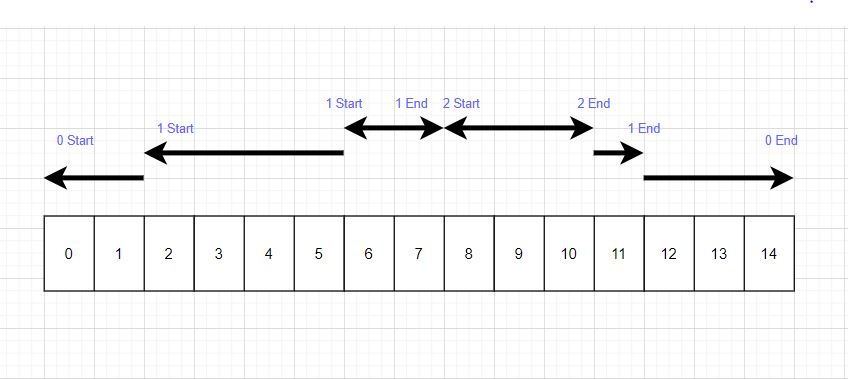New update is available. Click here to update.

# Execution Time

Last Updated: 21 Oct, 2020
Difficulty: Moderate

## PROBLEM STATEMENT

#### You are given a 2D array of integers containing information about ‘L’ logs where ith column represents the ith log message. Each column contains 3 rows to describe the ith log where,

``````1st Row - represents the ID of the function.

2nd Row - represents whether the function has started or ended where 1 denotes the start and -1 denotes the end.

3rd Row - represents the TIMESTAMP of the log.
``````

#### Note:

``````1. The exclusive time of a function is the sum of execution times for all calls of that function.

2. A function can be called multiple times, possibly recursively.

3. No two events will happen at the same time where an event denotes either a start or end of a function call. This basically means no two logs have the same timestamp.

4. Each function has an end log for each start log.
``````

#### For Example:

``````Consider the following input
0 1 1 1 2 2 1 0
1 1 1 -1 1 -1 -1 -1
0 2 5 7 8 10 11 14
````````````Thus, we return [5, 7, 3] as a process with ID 0 has taken 5 units of time and process with ID 1 has taken 7 units of time and process ID 2 has taken 3 units of time. A process’s exclusive time is the sum of exclusive times for all function calls in the program. As process Id 1 has called itself so exclusive time is the sum of exclusive times(5 + 2).
``````
##### Input Format:
``````The first line of input contains a single integer ‘T’, representing the number of test cases or queries to be run.
Then the T test cases follow.

The first line of each test case contains two single space-separated integers ‘N’ and ‘L’, representing the number of unique functions and number of logs respectively.

The second line of each test case contains ‘L’ single space-separated integers representing the function ID for each log.

The third line of each test case contains ‘L’ single space-separated integers(1 or -1), where 1 represent a function that has started execution and -1 represent a function that has ended the execution

The fourth line of each test case contains L single space-separated integers, representing the ‘TIMESTAMP.
``````
##### Output Format:
``````For each test case, print 'N' single space-separated integers, representing the exclusive time of each function in a single line.

The output of each test case will be printed in a separate line.
``````
##### Note:
``````You do not need to print anything, it has already been taken care of. Just implement the given function.
``````
##### Constraints:
``````1 <= T <= 10
1 <= N <= 10 ^ 2
2 <= L <= 5 * (10 ^ 2) and L is even.
0 <= TIMESTAMP <= 10 ^ 9

Time Limit: 1 sec.
``````## Approach 1

• Create a stack of integers that will store the unfinished function, top element will store the current function which is in execution.
• Push the first process ID into the stack.
• Initialize the ‘TIME’ to the timestamp of the first function.
• Create an array ‘ANS’ of ‘N’ size which will store the exclusive TIME of ith function at ith index.
• Traverse the whole array,
• Keep incrementing the exclusive TIME corresponding to the function on the top of the stack till the current timestamp equals the start/end timestamp corresponding to the next function.
• If the next function starts, then push it into the stack.
• Else, pop the front function ID.

Finally, return the ANS array.